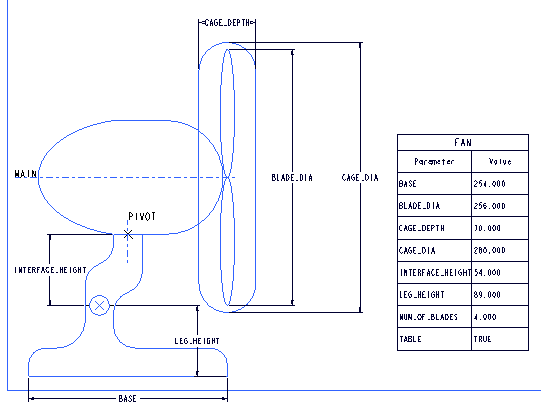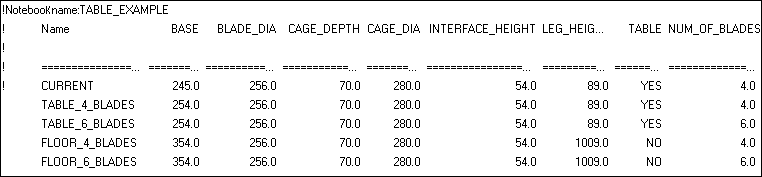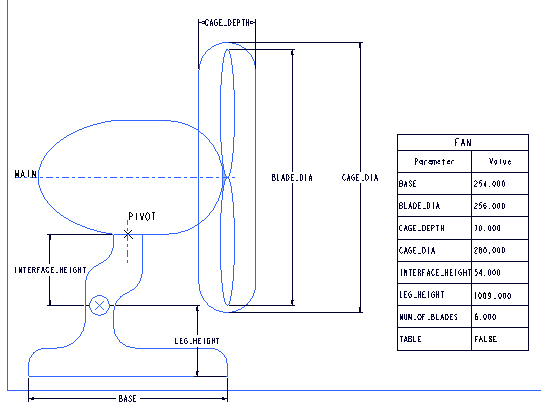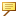Example: A Parameter Table
The following example shows the use of a Parameter Table in a notebook. In this example, the fan in the notebook can be a table model or a floor model with 4 or 6 blades. A Parameter Table determines the values for each set of parameters. When you choose a set of parameters and regenerate the notebook, the values in the notebook update to reflect those of the set.The following figure shows a Parameter Table created for the fan notebook.When you activate a different parameter set for the notebook, you must regenerate the notebook for it to be visible in the parameter table in the notebook sheet. In the figure below, the parameter set has been changed to Floor_6_blades.The notebook remains unchanged. A notebook is not parametric and does not update according to the parameter values.Parameters governed by a relation can be used in the Parameter Table.